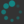In school we learn to compute the basic operations $$+,$$ $$-,$$ $$\times,$$ $$\div,$$ and perhaps $$\sqrt{\ \ }$$ by hand, and gain a visceral sense of how they work. When we later encounter more advanced functions—such as $$\cos,$$ $$\log,$$ or $$\arctan$$—these are treated as black boxes: we are told to simply "punch it into the calculator". I think this contributes to a sense of discomfort with these functions.

The goal of this unit is to learn to implement all the elementary functions on a computer, starting from first principles. This will also serve as an opportunity to review the theoretical properties of these functions; a recurring theme will be how theoretical properties can lead to practical algorithms. Finally, it will provide a concrete illustration of important concepts from analysis.